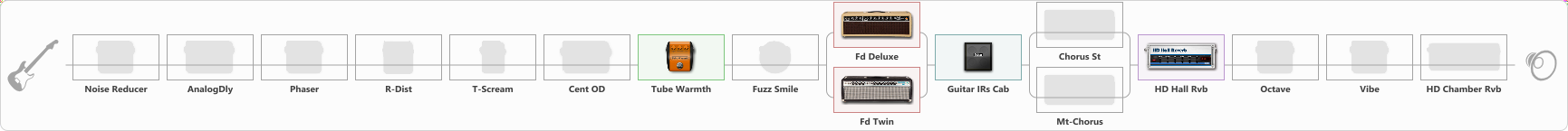# So natural sound for stratocaster

Discussion in 'ToneLib-GFX presets' started by jojo jotaro, Feb 18, 2023.

1. So natural sound for stratocaster

Preset name: User 1 GIMME THE DRIVE

Effects chain:Effect: "Noise Reducer" (Dynamics / Filter), active - "no"
{
"Sens" = 78
"Mode" = Soft
}

Effect: "AnalogDly" (Delay), active - "no"
{
"Time" = 388
"Feedback" = 43
"Tone" = 55
"Mix" = 19
}

Effect: "Phaser" (Modulation / Sfx), active - "no"
{
"Model" = MXR Phase 100
"Speed" = 8.3
"Depth" = 7
"Reso" = 27
"Freq" = 8.0
}

Effect: "R-Dist" (Overdrive / Distortion), active - "no"
{
"Drive" = 56
"Tone" = 56
"Level" = 71
}

Effect: "T-Scream" (Overdrive / Distortion), active - "no"
{
"Drive" = 84
"Tone" = 40
"Level" = 86
}

Effect: "Cent OD" (Overdrive / Distortion), active - "no"
{
"Drive" = 44
"Tone" = 51
"Level" = 72
}

Effect: "Tube Warmth" (Dynamics / Filter), active - "yes"
{
"Input" = 72
"Warmth" = 58
"Level" = 53
}

Effect: "Fuzz Smile" (Overdrive / Distortion), active - "no"
{
"Drive" = 20
"Tone" = 41
"Level" = 90
}

Effect: "Splitter" (Dynamics / Filter)
{
"A-Bypass" = Off
"A-Pan" = -25
"A-Level" = 55
"B-Bypass" = Off
"B-Pan" = 25
"B-Level" = 55
"Width" = 0

'A' branch:
{

Effect: "Fd Deluxe" (Amp simulators), active - "yes"
{
"Gain" = 25
"Bass" = 49
"Middle" = 58
"Treble" = 50
"Presence" = 80
"Master" = 100
"Level (dB)" = 0
}
}
'B' branch:
{

Effect: "Fd Twin" (Amp simulators), active - "yes"
{
"Gain" = 24
"Bass" = 42
"Middle" = 58
"Treble" = 47
"Presence" = 19
"Master" = 79
"Level (dB)" = 0
}
}
}

Effect: "Guitar IRs Cab" (Cabinets), active - "yes"
{
"Model" = Marshall 1960b (4x12")
"Mic Position" = Center
"Mic Distance" = Near
"Low Cut (Hz)" = 43
"Hi Cut (kHz)" = 8.0
"Mix" = 77
"Level (dB)" = 14
}

Effect: "Splitter" (Dynamics / Filter)
{
"A-Bypass" = Off
"A-Pan" = -25
"A-Level" = 55
"B-Bypass" = Off
"B-Pan" = 25
"B-Level" = 55
"Width" = 0

'A' branch:
{

Effect: "Chorus St" (Modulation / Sfx), active - "no"
{
"Speed" = 4.7
"Depth" = 64
"Center" = 3.2
"Mix" = 40
}
}
'B' branch:
{

Effect: "Mt-Chorus" (Modulation / Sfx), active - "no"
{
"Speed" = 4.8
"Depth" = 56
"Time" = 1.7
"Mix" = 26
}
}
}

Effect: "HD Hall Rvb" (Reverberation), active - "yes"
{
"Time" = 7.7
"PreLPF" = 28
"PreDelay" = 49
"HiDamp" = 13
"LoGain" = -1.9
"Mix" = 8
}

Effect: "Octave" (Modulation / Sfx), active - "no"
{
"Direct" = 79
"-1 Oct" = 13
"-2 Oct" = 0
}

Effect: "Vibe" (Modulation / Sfx), active - "no"
{
"Speed" = 7.3
"Depth" = 21
"Mix" = 9
}

Effect: "HD Chamber Rvb" (Reverberation), active - "no"
{
"Time" = 5.8
"PreLPF" = 85
"PreDelay" = 61
"HiDamp" = 53
"LoGain" = 12.0
"Mix" = 24
}

Note: You will need to download and install the ToneLib-GFX software to use the preset.

File size:
1.6 KB
Views:
3,072